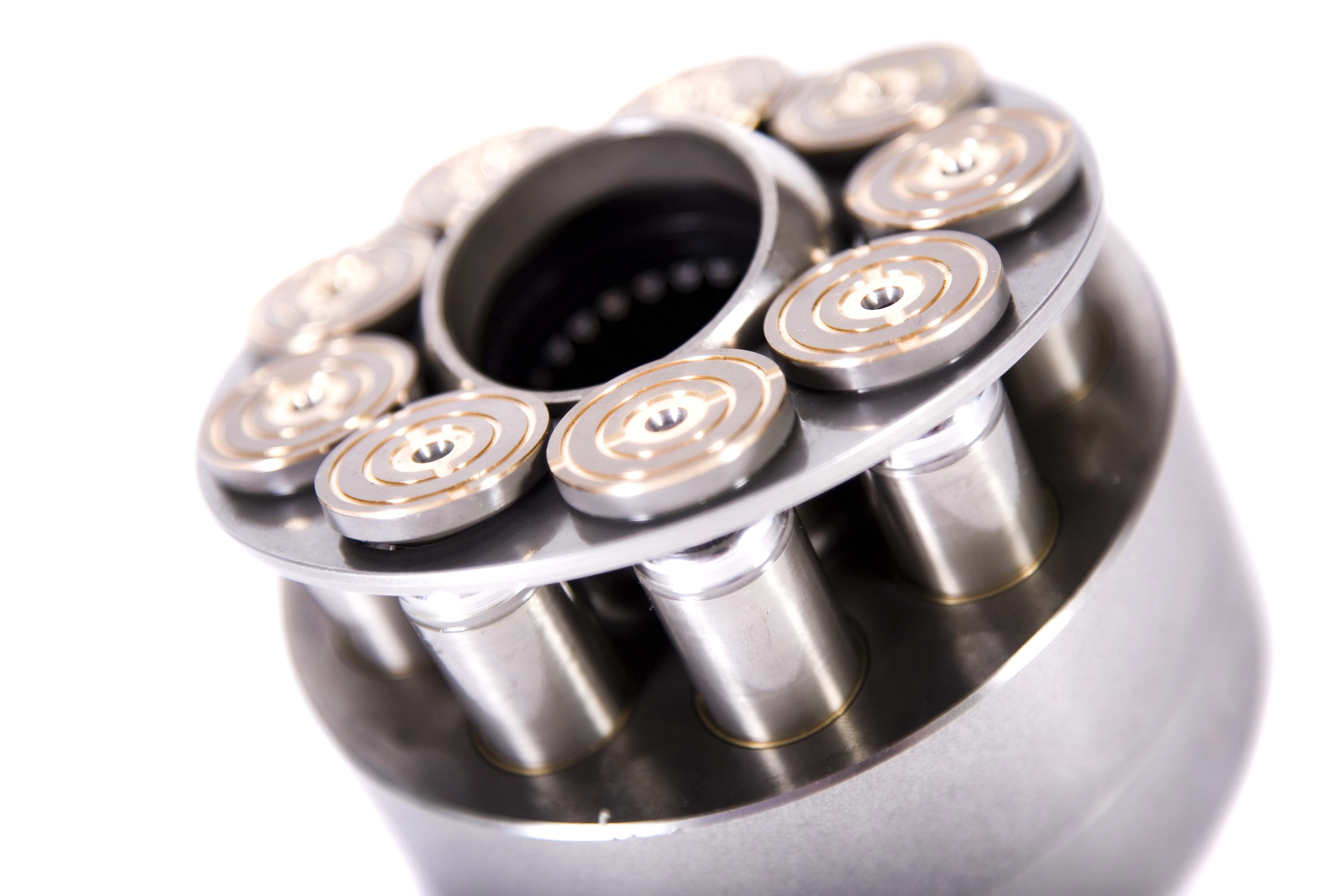Local time: Fri 12 Jun 2020 05:40<h1 class="h2">Blog</h1>

<div class="__stroke expanded">&nbsp;</div>
</div>

# Basic Hydraulics and How to Calculate Hydraulic System Pressure

There are specific calculations you can perform when you want to find out what the most appropriate hydraulic system pressure is for a particular application. Before we discuss the formulas used to determine pressure, let’s do a quick review of some hydraulic basics.

Hydraulic pumps are designed to produce a flow of fluid through the system. Pressure in the system occurs as a result of any resistance to the flow of the fluid. According to Pascal’s Law, when a force is applied at any point in a confined system, fluid is transmitted in all directions at the same pressure.

As a result, cylinders within the system will all move at their own rate based on the weight of the load being moved. To illustrate, cylinders with the lighter weights will start to lift first, followed by the next heaviest, and so on. In order to get cylinders with different weight loads to move simultaneously, pressure control valves or other such components must be added to the system.

However, you will need to calculate how much pressure each cylinder requires to extend. The basic equation to use is F (Force) = P (Pressure) x A (Surface Area). You can rearrange the equation to solve for pressure using P = F/A.

• TIP: There are online calculators available, where you simply plug in the values to get the result.

For assistance in determining system pressure and all of your hydraulic cylinders and system parts and components, please contact White House Products Ltd. at +44 (0) 1475 742500 today!

Back to blog posts